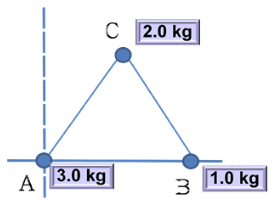# Problem: Three particles of mass 3.0 kg, 1.0 kg, and 2.0 kg are placed at the vertices A, B, C, of an equilateral triangle ABC of edge 2.0 m. (1) Find x coordinate of the center of mass (XCN) of three-particle system shown in the figure.

###### FREE Expert Solution

X-coordinate of the center of mass:

$\overline{){{\mathbf{x}}}_{\mathbf{c}\mathbf{m}}{\mathbf{=}}\frac{{\mathbf{m}}_{\mathbf{1}}{\mathbf{x}}_{\mathbf{1}}\mathbf{+}{\mathbf{m}}_{\mathbf{2}}{\mathbf{x}}_{\mathbf{2}}\mathbf{+}\mathbf{.}\mathbf{.}\mathbf{.}\mathbf{+}{\mathbf{m}}_{\mathbf{n}}{\mathbf{x}}_{\mathbf{n}}}{{\mathbf{m}}_{\mathbf{1}}\mathbf{+}{\mathbf{m}}_{\mathbf{2}}\mathbf{+}\mathbf{.}\mathbf{.}\mathbf{.}\mathbf{+}{\mathbf{m}}_{\mathbf{n}}}}$

100% (182 ratings)###### Problem Details

Three particles of mass 3.0 kg, 1.0 kg, and 2.0 kg are placed at the vertices A, B, C, of an equilateral triangle ABC of edge 2.0 m.(1) Find x coordinate of the center of mass (XCN) of three-particle system shown in the figure.